×
Get Full Access to Physics: Principles With Applications - 6 Edition - Chapter 2 - Problem 21q
Get Full Access to Physics: Principles With Applications - 6 Edition - Chapter 2 - Problem 21q

×

Describe in words the motion plotted in Fig. 2-32 in termsISBN: 9780130606204 3

Solution for problem 21Q Chapter 2

Physics: Principles with Applications | 6th Edition

• Textbook Solutions
• 2901 Step-by-step solutions solved by professors and subject experts
• Get 24/7 help from StudySoup virtual teaching assistantsPhysics: Principles with Applications | 6th Edition

4 5 1 254 Reviews
20
1
Problem 21Q

Describe in words the motion plotted in Fig.in terms of, etc. [Hint: First try to duplicate the motion plotted by walking or moving your hand.]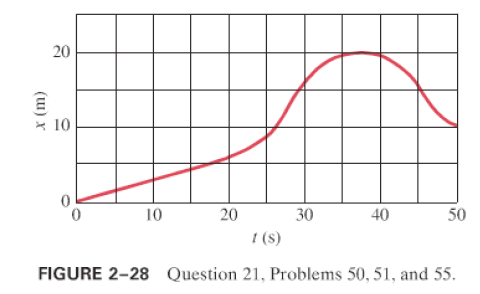Step-by-Step Solution:

Step 1 of 3

We will describe the plotted graph of a motion above.

But we need to know what how to read the speed from the graph

Slope

The slope of x-t graph of a motion gives velocity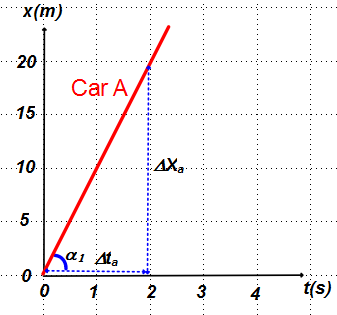The x-t graph of car A is given aside. The slope of the graph gives its velocity.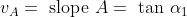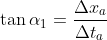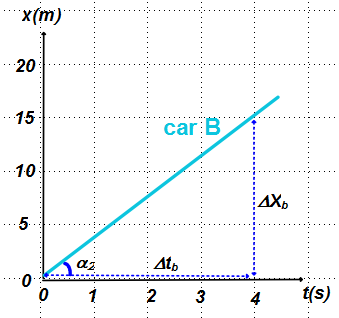The x-t graph of car B is given aside.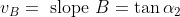The slope of car A is bigger than slope of car B.  So velocity of car A is bigger than car B. In both two motions above, the velocity of the objects are constant , the slopes of the graphs are constant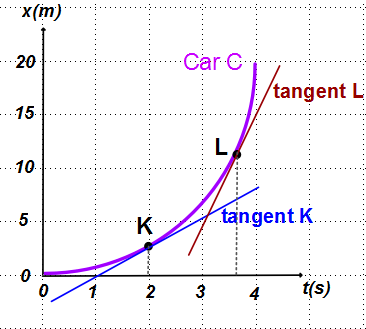The x-t graph of car C is given aside. It is a curve, so its slope is changing. If slopes changes velocity changes.

The slope of the line tangent to graph at point gives velocity of object at that point._______________

Step 2 of 3

Step 3 of 3

ISBN: 9780130606204

Unlock Textbook Solution